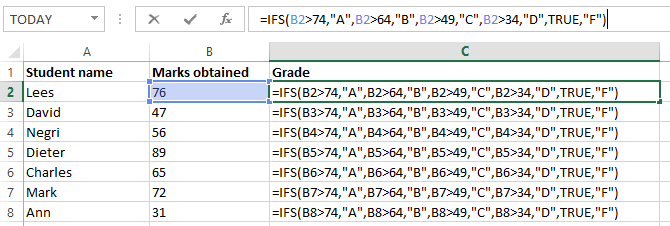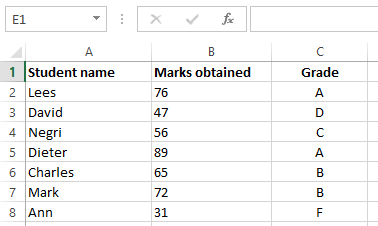# How To Use IFS Function In ExcelIFS function is a new function added to Excel and only available in the latest version of Office (EXCEL 2016, Excel Online and latest mobile excel versions). Therefore when opening an IFS function containing excel workbook in an earlier version of excel, IFS function containing cells will be shown as #NAME? error notification and become unusable.

The basic function of this is to check pre-defined conditions and then return a corresponding value which are specified by the user.

The syntax used is explained below:

IFS(logical_test1, value_if_true1, [logical_test2, value_if_true2], [logical_test3, value_if_true3],…)

logical_test1:                    The conditional argument 1, which would be evaluated as TRUE or FALSE. (Required)

value_if_true1:                The value to be returned if the condition 1 (i.e. logical_test1) is true. This can be left empty.

logical_test2:                    The conditional argument 2, which would be evaluated as TRUE or FALSE. (Optional)

value_if_true2:                The value to be returned if the condition 2 (i.e. logical_test2) is true. This can be left empty.

Note: Up to 127 conditions can be added to this function, following the same argument style.

Example #1:

Consider the following data set, the IFS function has been used to assign grades for exam marks.The used syntax is explained below.

=IFS(B2>74,"A",B2>64,"B",B2>49,"C",B2>34,"D",TRUE,"F")

B2>74,"A" – if B2 is greater than 74, then it will return “A”

B2>64,"B" – if B2 is greater than 64, then it will return “B”

B2>49,"C" – if B2 is greater than 49, then it will return “C”

B2>34,"D" – if B2 is greater than 35, then it will return “D”

TRUE,"F") – the values below 35 will not meet any of the above conditions, so it would be considered as ‘TRUE’ and then it will return “F”Note: The same result can be obtained using the Nested IF function. But the IFS function allows to perform it in a much easier and compact way.

Tips:

• If a logical_test is entered without specifying a return value (i.e. value_if_true), the function will return an error message “You've entered too few arguments for this function”.

• If the function is unable find a ‘TRUE’ condition, then #N/A error would be returned.